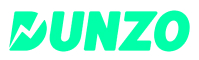New update is available. Click here to update.
Topics

# Serialize and Deserialize Binary Tree

Hard0/120
Average time to solve is 15m+11 more companies

## Problem statement

You have been given a binary tree of integers. You are supposed to serialize and deserialize (refer to notes) the given binary tree.

You can choose any algorithm to serialize/deserialize the given binary tree. You only have to ensure that the serialized string can be deserialized to the original binary tree.

Note :
``````Serialization is the process of translating a data structure or object state into a format that can be stored or transmitted (for example, across a computer network) and reconstructed later. The opposite operation, that is, extracting a data structure from stored information, is deserialization.
``````
Detailed explanation ( Input/output format, Notes, Images )
Sample Input 1 :
``````1 -1 3 -1 -1
``````
Sample Output 1 :
``````1 -1 3 -1 -1
``````
Explanation For Sample Input 1 :
``````The given tree looks as follows:
1
\
3
The level order traversal of the given tree will be “1 -1 3 -1 -1” where -1 denotes the null nodes.
``````
Sample Input 2 :
``````1 2 3 -1 4 5 -1 -1 -1 -1 -1
``````
Sample Output 2 :
``````1 2 3 -1 4 5 -1 -1 -1 -1 -1
``````
Explanation For Sample Input 2:
``````The given tree looks as follows:
1
/ \
2   3
\   /
4 5

The level order traversal of the given tree will be “1 2 3 -1 4 5 -1 -1 -1 -1 -1" where -1 denotes the null nodes.
``````
Expected time complexity:
``````The expected time complexity is O(n).
``````
Constraints :
``````0 <= 'n' <= 10^6
1 <= 'data' <= 10^8 and data != -1,
Where 'n' is the number of nodes and 'data' is the value at the nodes.

Time Limit: 1 sec
``````Console